# Boundary Layer Analysis Adjacent to Moving Heated Plate Inside Electrically Conducting Fluid with Heat Source/Sink

Boundary Layer Analysis Adjacent to Moving Heated Plate Inside Electrically Conducting Fluid with Heat Source/Sink
Department of Physics and Engineering Mathematics, Faculty of Engineering, Zagazig University, Zagazig 44519, Egypt

Delta Higher Institute for Engineering and Technology, Mansoura 35681, Egypt

Corresponding Author Email:
asrashed@zu.edu.eg
Page:
682-688
|
DOI:
https://doi.org/10.18280/ijht.380312
12 November 2019
|
Accepted:
24 July 2020
|
Published:
15 October 2020
| Citation

OPEN ACCESS

Abstract:

Newtonian steady state flow of fluids with electrical conduction properties was examined adjacent to a moving heated vertical plate subjected to a magnetic field and a heat source/sink. The impact of magnetic parameter, Prandtl number, permeability coefficient, heat source/sink volumetric rate and temperature difference between heated plate and ambient temperature. A reduced system of ODEs was created via group similarity method. The solution led to some important results.  Increasing permeability coefficient of the plate material resulted in a significant increase in flow velocity and a slight increase in heat flux but the magnitude of shear stress and temperature distribution decreased. Moreover, increasing the magnetic parameter, M, led to a significant decrease in velocity and a decrease in heat flux, whereas shear stress and temperature distribution increased. Furthermore, increasing Prandtl number, Pr, reduced the velocity significantly and the heat flux slightly. On the other hand, the magnitude of shear stress and temperature distribution increased. In case of using heat source, the increase in its energy rate decreased the heat flux with no significant effect on shear stress. Finally, the increment of temperature difference led to noticeable increase in velocity and a slight increase in heat flux, whereas the shear stress decreased.

Keywords:

electrically conducting fluids, group method, magnetic parameter, Prandtl number

1. Introduction

For many decades, Newtonian flows attracts many researchers to investigate and study their behaviors.  Steady and unsteady fluid dynamics were studied for different cases of operations to model and simulate many engineering applications. Power generators, cooling systems of nuclear reactors and liquid metal flow control are few examples of such applications. Electrically conducting fluids subjected to magnetic field, which are also known as magnetohydrodynamic (MHD) fluids, are important fluids models. Many researchers have studied different cases of MHD fluids using numerous methods. Kataria and Patel  studied the effects of heat generation on MHD fluid flow through porous medium past an oscillating vertical plate. Huang and Liu , analyzed the features of laminar MHD fluid in a pipe. Liu and Guo  examined the fractional Maxwell MHD fluid. Ahmad et al.  used a periodically accelerated plate to find a new analytical technique for MHD fluid flow. Ajam et al.  used Buongiorno’s model and found a new analytical approximation of MHD resulting from a stretching permeable surface. Chen et al.  obtained a solution for fractional viscoelastic MHD fluid using Lie group similarity over a stretching sheet. Khan et al.  attained a numerical solution of MHD flow with homogenous heterogeneous reactions. Prasad et al.  studied the thermal properties of MHD Casson fluid. Umavathi et al.  studied the effect of temperature on MHD flow in a vertical channel. Ahmed et al.  investigated the non-Newtonian Maxwell fluid with variable thermal conductivity. Rehman et al.  studied MHD flow of Casson fluid in stretching cylinder. Different mathematical methods were exploited to investigate and analyze numerous cases of fluid dynamics. Lie Infinitesimal and group methods [12-14], homotopy method [15-17], finite element [18-20] and finite volume [21-23] are examples for such common methods. Several modeling of MHD fluids have been studied [24, 25].

Inspired by all these researches, the present work provides analytical and numerical solutions for heated moving vertical plate submerged in MHD fluid. The objectives of the recent study are to combine many parameters to the considered flow and investigate their effect on the velocity profile, shear stress, heat distribution and heat flux inside the boundary layer. The considered parameters are magnetic parameter, permeability coefficient, Prandtl number, temperature difference and volumetric heat rate of a heat source/sink.

2. Mathematical Formulation

Consider a moving vertical porous plate immersed in MHD fluid with temperature adjacent to plate of Tw while temperature outside boundary layer is $T_{\infty}$. The flow undergoes a constant pressure and subjected to a constant magnetic field of density B0 in y-direction that results in Lorentz force in x-direction $\left(-\sigma B_{0}^{2} u\right)$. The momentum in y-direction has been neglected while the heat diffusion is more significant in y-direction. Based on the previous assumptions, the physical model is depicted in Figure 1 while the governing equations are described as:

## 1.pngFigure 1. Physical model of the problem

$\frac{\partial u}{\partial x}+\frac{\partial v}{\partial y}=0$     (1)

$u \frac{\partial u}{\partial x}+v \frac{\partial u}{\partial y}=v \frac{\partial^{2} u}{\partial y^{2}}+g \beta\left(T-T_{\infty}\right)-\frac{\sigma}{\rho} B_{0}^{2} u-\frac{v}{k} u$    (2)

$u \frac{\partial T}{\partial x}+v \frac{\partial T}{\partial y}=\alpha \frac{\partial^{2} T}{\partial y^{2}}+\frac{16 \sigma^{*}}{3 \rho c_{p} \chi} T_{\infty}^{3} \frac{\partial^{2} T}{\partial y^{2}}+\frac{Q}{\rho c_{p}}\left(T-T_{\infty}\right)$      (3)

where, v is the kinematic viscosity, T is the temperature, k stands for permeability while $\alpha$ is the thermal diffusivity $\alpha=\frac{v}{P r}$ (Pr is Prandtl number).

The flow is Subjected to the following boundary conditions:

$u(x, 0)=u_{w}(x), u(x, \infty)=0, v(x, 0)=0,\\T(x, 0)=T_{\infty}$      (4)

The equations, Eq. (1) - Eq. (3), are normalized using the following substitutions:

$U(x, y)=\frac{u(x, y)}{u_{w}(x, y)}$     (5)

$\theta=\frac{T-T_{\infty}}{T_{w}-T_{\infty}}$      (6)

The equations, Eq. (1) - Eq. (3), are transformed to:

$U \frac{d u_{w}}{d x}+u_{w} \frac{\partial U}{\partial x}+\frac{\partial V}{\partial y}=0$     (7)

$U u_{w}\left(U \frac{d u_{w}}{d x}+u_{w} \frac{\partial U}{\partial x}\right)+v u_{w} \frac{\partial U}{\partial y}$ $-v u_{w} \frac{\partial^{2} U}{\partial y^{2}}-g \beta \theta \Delta T-\frac{\sigma}{\rho} B_{0}^{2} U u_{w}+\frac{v}{k} U u_{w}=0$      (8)

$U u_{w} \frac{\partial \theta}{\partial x}+v \frac{\partial \theta}{\partial y}-\alpha \frac{\partial^{2} \theta}{\partial y^{2}}-\frac{16 \sigma^{*} T_{\infty}^{3}}{3 \rho c_{p} \chi} \frac{\partial^{2} \theta}{\partial y^{2}}-\frac{q}{\rho c_{p}} \theta=0$     (9)

The boundary conditions are:

$U(x, 0)=1, v(x, 0)=0, \theta(x, 0)=1$ $U(x, \infty)=0, \theta(x, \infty)=0$     (10)

3. Group Transformation of MHD System

A group transformation of one parameter, a, was used to reduce the PDE system into an ODE system in one similarity variable, $\eta$.

3.1 Formulation of the problem using group method

The group structure is assumed to be on the form [12, 13]:

$G: \bar{S}=K^{s}(a) S+Q^{s}(a)$    (11)

where, S stands for the system variables. The differential coefficients function, $K^{S}$ and $Q^{s}$, are real constants. The partial derivatives are defined as:

$\left. \begin{matrix} {{{\bar{S}}}_{{\bar{\iota }}}}=(\frac{{{k}^{s}}}{{{k}^{i}}}){{S}_{i}} \\ {{{\bar{S}}}_{\overline{\iota J}}}=(\frac{{{k}^{s}}}{{{k}^{i}}{{k}^{j}}}){{S}_{i}}_{j} \\\end{matrix} \right\}i=x,y\text{ and j=x,y}$  (12)

3.2 Analysis of the problem

Eqns. (7) - (9) are transformed to:

$\bar{U} \frac{d \bar{u}_{w}}{d \bar{x}}+\bar{u}_{w} \frac{\partial \bar{U}}{\partial \bar{x}}+\frac{\partial \bar{v}}{\partial \bar{y}}=H_{1}(a)\left[U \frac{d u_{w}}{d x}+u_{w} \frac{\partial U}{\partial x}+\frac{\partial v}{\partial y}\right]$      (13)

$\bar{U} \bar{u}_{w}\left(\bar{U} \frac{d \bar{u}_{w}}{d \bar{x}}+\bar{u}_{w} \frac{\partial \bar{U}}{\partial \bar{x}}\right)+\bar{v} \bar{u}_{w} \frac{\partial \bar{v}}{\partial \bar{y}}-v \bar{u}_{w} \frac{\partial^{2} \bar{U}}{\partial \bar{y}^{2}}-$$g \beta \bar{\theta} \Delta T-\frac{\sigma}{\rho} B_{0}^{2} \bar{U} \bar{u}_{w}+\frac{v}{K} \bar{U} \bar{u}_{w}$$=H_{2}(a)\left[U u_{w}\left(U \frac{d u_{w}}{d x}+u_{w} \frac{\partial U}{\partial x}\right)+v u_{w} \frac{\partial U}{\partial y}-\right.$$\left.v u_{w} \frac{\partial^{2} U}{\partial y^{2}}-g \beta \theta \Delta T-\frac{\sigma}{\rho} B_{0}^{2} U u_{w}+\frac{v}{k} U u_{w}\right] (14) \bar{U} \bar{u}_{w} \frac{\partial \bar{\theta}}{\partial \bar{x}}+\bar{v} \frac{\partial \bar{\theta}}{\partial \bar{y}}-\alpha \frac{\partial^{2} \bar{\theta}}{\partial \bar{y}^{2}}-\frac{16 \sigma^{*} T_{\infty}^{3}}{3 \rho c_{p} \chi} \frac{\partial^{2} \bar{\theta}}{\partial \bar{y}^{2}}-\frac{q}{\rho c_{p}} \bar{\theta}$$=H_{3}(a)\left[U u_{w} \frac{\partial \theta}{\partial x}+v \frac{\partial \theta}{\partial y}-\alpha \frac{\partial^{2} \theta}{\partial y^{2}}-\frac{16 \sigma^{*} T_{\infty}^{3}}{3 \rho c_{p} \chi} \frac{\partial^{2} \theta}{\partial y^{2}}-\right.$$\left.\frac{q}{\rho c_{p}} \theta\right]$       (15)

Invariance condition [12, 13] for Eq. (13)-Eq. (15) leads to:

$K^{y}=K^{v}=1, K^{x}=K^{U} K^{u_{w}}, K^{\theta}=K^{x}$      (16)

$Q^{U}=Q^{u_{w}}=Q^{v}=Q^{\theta}=0$     (17)

Finally, the full group structure can be described as:

G:\left\{ \begin{matrix} {{G}_{1}}\left\{ \begin{matrix} \overline{x}={{K}^{x}}x+{{Q}^{x}} \\ \overline{y}=y+{{Q}^{y}} \\\end{matrix} \right. \\ {{G}_{2}}\left\{ \begin{matrix} \overline{U}={{K}^{U}}U \\ \begin{align} & \overline{{{u}}}_{w}=\frac{{{k}^{x}}}{{{k}^{{{u}_{w}}}}}{{u}_{w}} \\ & \overline{v}=V \\ & \overline{\theta}{ ={{K}^{x}}\theta } \\\end{align} \\\end{matrix} \right. \\\end{matrix} \right.   (18)

3.3 The complete transform of MHD system

Recalling Morgan's theorem for group method of one parameter, the independent variables can be reduced into one similarity variable. Moreover, the dependent variables, $U, u_{w}, v$ and $\theta$ are transformed into new invariant variables. Morgan’s theorem states:

$\sum_{i=1}^{6}\left(\gamma_{i} S_{i}+\delta_{i}\right) \frac{\partial q_{i}}{\partial s_{i}}=0$     (19)

where, $S_{i}$  refers to the original system variables ( $x, y, U, u_{w}, V, \theta$) and $q_{i}$ refers to the transformed variables while the $\gamma_{i}$ and $\delta_{i}$ are defined as:

$\left\{\begin{array}{l}\gamma_{i}=\frac{\partial K^{S} i(a)}{\partial a} \\ \delta_{i}=\frac{\partial Q^{S_{i}(a)}}{\partial a}\end{array}\right.$    （20）

3.3.1 Transformation of the independent variables

Applying Eq. (19) to the original independent variables, helps in attaining a new similarity variable in the form:

$\eta(x, y)=y \Gamma(x)$    (21)

whereas the dependent variables are transformed to new invariant variables in the form:

$\left\{\begin{array}{l}U=\omega(x) F(\eta) \\ u_{w}=u_{w}(x) \\ v=V(\eta) \\ \theta=\pi(x) E(x)\end{array}\right.$    (22)

where, $\Gamma(x), \omega(x), u_{w}(x)$ and $\pi(x)$ are arbitrary functions which will be evaluated during the reduction process of the system. The system, Eq. (7) - Eq. (9), will be reduced to the following system where dashes indicate derivatives with respect to $\eta$:

$V^{\prime}+\left(v^{2}+\frac{a-v^{3}}{v}\right) F=0$     （23）

$v^{2} F^{2}+\left(\frac{a-v^{3}}{v}\right) F^{2}+v F^{\prime}$

$=F^{\prime \prime}+g \beta \Delta T E-\left(\frac{M}{v}+\frac{1}{K}\right) v^{2} F$     (24)

$a E F+v V E^{\prime}=\left(\frac{v}{P r}+H\right) E^{\prime \prime}+\frac{q v^{2}}{\rho c_{p}} E$     (25)

Such that the arbitrary functions were evaluated to be:

$\Gamma(x)=\frac{1}{v}, \eta(x, y)=\frac{y}{v}, \pi(x)=\frac{c_{0}}{v^{3}} x+c_{1}$,$\omega(x)=c_{2}\left(\frac{c_{0}}{v^{3}} x+c_{1}\right)^{\frac{v^{3}}{a}}, u_{w}=\frac{v}{c_{2}}\left(\frac{c_{0}}{v^{3}} x+c_{1}\right)^{\frac{c_{0}-v^{3}}{c_{0}}}$     (26)

where, $M=\frac{\sigma}{\rho} B_{0}^{2}, H=\frac{16 \sigma^{*}}{3 \rho c_{p} \chi} T_{\infty}^{3}$. Moreover, $c_{0}, c_{1}$ and $c_{2}$ are arbitrary constants.

The obtained ODE system is subjected to the following conditions:

$F(0)=1, V(0)=0, E(0)=1$,$F(\infty)=0, E(\infty)=0$      (27)

4. Results and Discussion

Eqns. (23) - (25) are numerically solved using shooting method. The effects of Prandtl number, Pr, magnetic parameter, M, permeability coefficient of the plate, k, the volumetric heat rate of the source/sink, q, and temperature difference, $\Delta T$, were investigated for MHD flow.

4.1 Effect of permeability coefficient, k

The results showed that, increasing permeability coefficient of the plate material led to a corresponding significant increase in the fluid velocity and a slight increase in the heat flux inside the boundary layer. On the other hand, the magnitude of shear stress and temperature distribution decreased with increasing permeability coefficient. This can be explained by the fact that the pores in porous plate facilitated the flow diffusion and heat flux as shown in Figures 2-5.

4.2 Effect of magnetic parameter, M

The results showed that, increasing magnetic parameter, M, or increasing the magnetic flux density led to a corresponding significant decrease in the fluid velocity and a slight decrease in the heat flux inside the boundary layer. This is due to the retarding effect of Lorentz magnetic forces on the fluid diffusion. On the other hand, the magnitude of shear stress and temperature distribution increased with increasing M values as illustrated in Figures 6-9.

4.3 Effect of Prandtl number, Pr

The results showed that, increasing Prandtl number, Pr, or increasing the viscosity of the fluid led to a corresponding significant decrease in flow velocity and a slight decrease in the heat flux inside the boundary layer. This is due to the effect of increasing fluid viscosity on retarding the fluid flow. On the other hand, the magnitude of shear stress and temperature distribution increase with increasing Pr values as illustrated in Figures 10-13.

## 2.png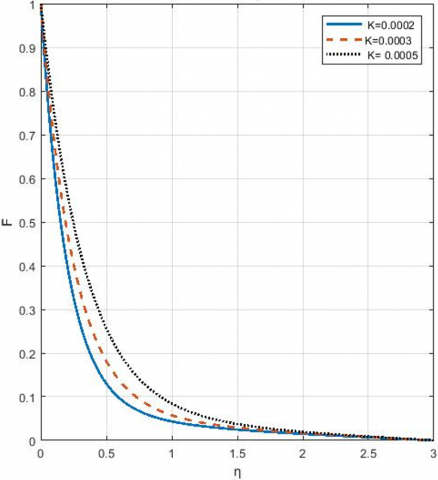Figure 2. Effect of permeability coefficient on velocity

## 3.png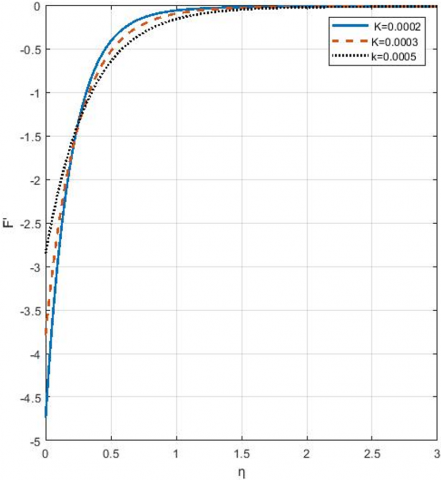Figure 3. Effect of permeability coefficient on shear stress

## 4.png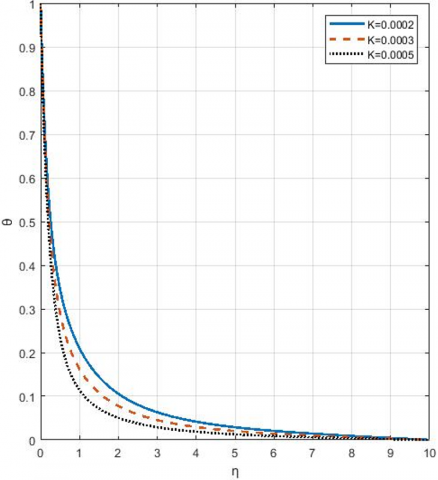Figure 4. Effect of permeability coefficient on temperature distribution

## 5.png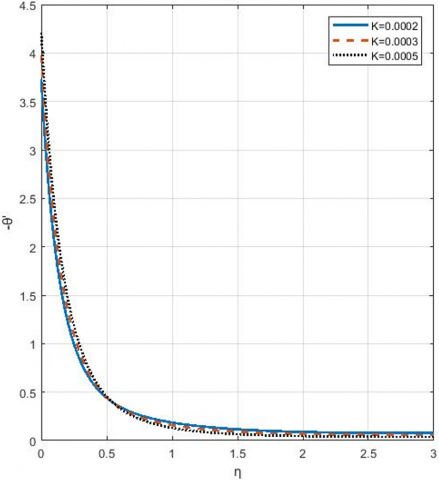Figure 5. Effect of permeability coefficient on heat flux

## 6.png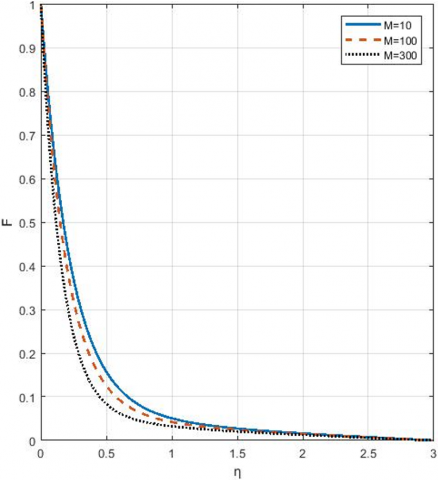Figure 6. Effect of magnetic parameter on velocity

## 7.png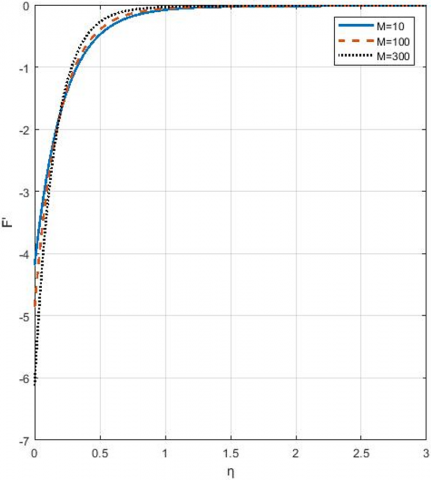Figure 7. Effect of magnetic parameter on shear stress

## 8.pngFigure 8. Effect of magnetic parameter on temperature

## 9.png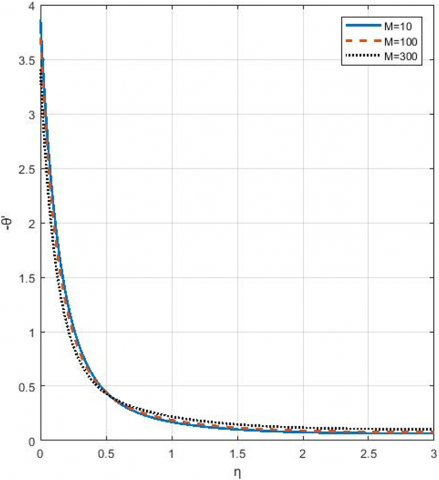Figure 9. Effect of magnetic parameter on heat flux

## 10.pngFigure 10. Effect of Prandtl number on velocity

## 11.png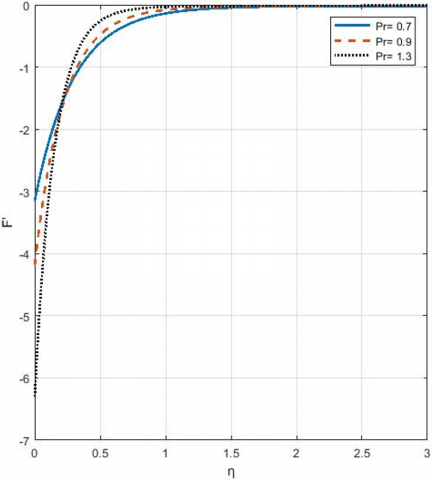Figure 11. Effect of Prandtl number on shear stress

## 12.pngFigure 12. Effect of Prandtl number on temperature

## 13.png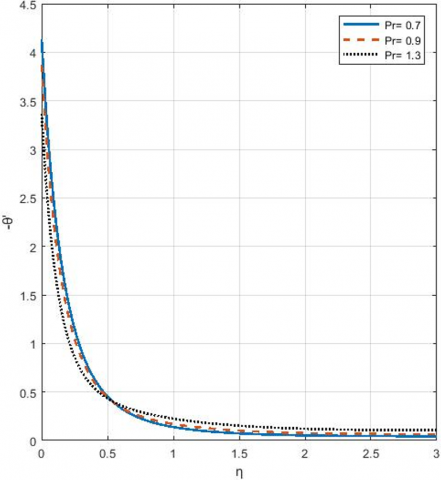Figure 13. Effect of Prandtl number on heat flux

4.4 Effect of source/sink volumetric heat rate, q

The results showed that, increasing source energy from 0.2 to 1 slightly increased the fluid velocity and slightly decreased the heat flux, whereas it had no significant effect on shear stress. Moreover, increasing sink energy from -2 to -3 had a slight effect on the velocity and heat flux while it had no significant effect on shear stress as shown in Figures 14-16.

4.5 Effect of temperature difference, $\left(\Delta T=T_{w}-T_{\infty}\right)$

Also, the results showed that, increasing $\Delta T$  led to a corresponding significant increase in the fluid velocity and a slight increase in the heat flux inside the boundary layer. This is due to the energy gained by the fluid which activated the fluid particles. On the other hand, the magnitude of shear stress decreased with increasing $\Delta T$  values as illustrated in Figures 17-19.

## 14.png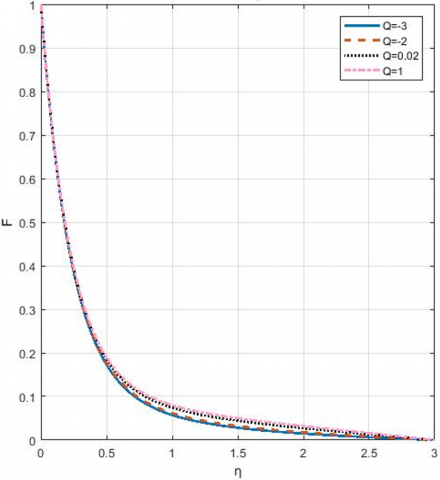Figure 14. Effect of source/sink heat rate energy on velocity

## 16.png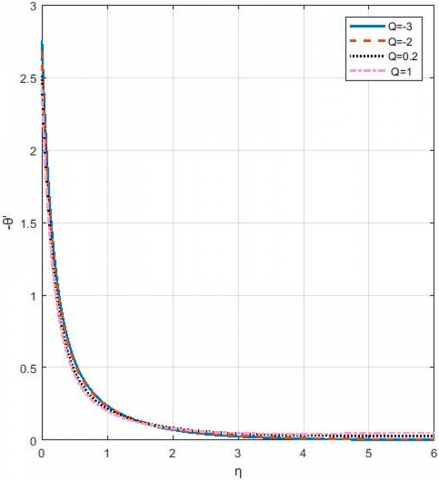## 15.png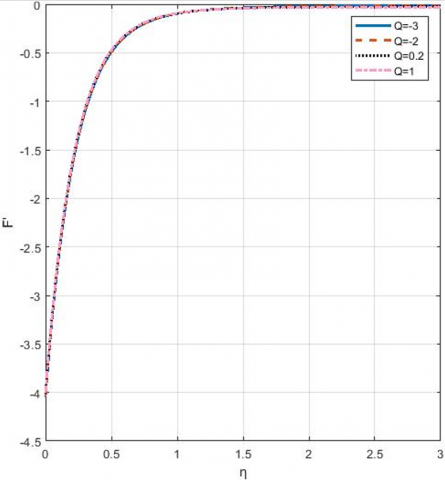Figure 15. Effect of source/sink heat rate energy on shear stress

## 16.pngFigure 16. Effect of source/sink heat rate energy on heat flux

## 17.pngFigure 17. Effect of temperature difference between the plate and ambient temperature on velocity

## 18.png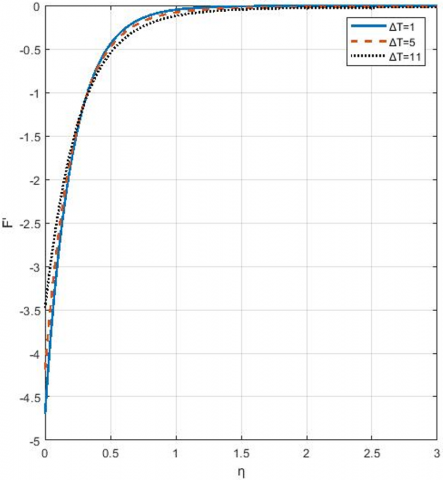Figure 18. Effect of temperature difference between the plate and ambient temperature on shear stress

## 19.png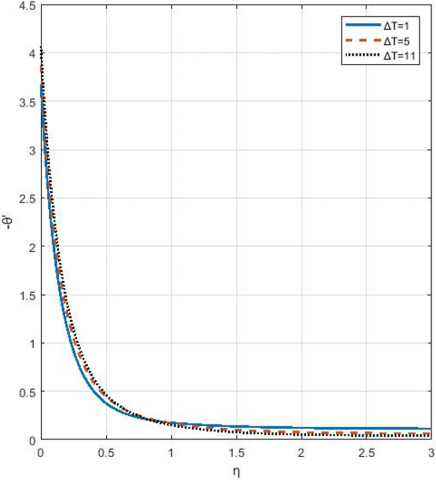Figure 19. Effect of temperature difference between the plate and ambient temperature on heat flux profile

5. Conclusions

Investigation and analysis of electrically conducting fluid containing a moving vertical plate has been executed in the presence of external magnetic field and heat source/sink. The following results have been obtained

(1) Increasing the permeability coefficient, k, of the plate material increases the fluid velocity and heat flux, significantly, whereas it decreases the shear stress and temperature distribution.

(2) If a heat source exists, the increment in its volumetric heat rate, q, slightly increases the fluid velocity, but slightly decreases heat flux with no significant effect on shear stress.

(3) If a heat sink exists, the increment in its volumetric heat rate, q, has a slight effect on the fluid velocity and heat flux, but it has no noticeable effect on shear stress.

(4) The temperature difference between the heated plate and ambient temperature outside the boundary layer increases the fluid velocity and the heat flux. On the contrary, it decreases the shear stress and temperature distribution.

(5) Increasing the magnetic parameter, M, and Prandtl number, Pr, decrease velocity and heat flux due to the Lorentz forces and fluid viscosity increment, respectively, which obstruct the fluid particles. The opposite behavior occurs with shear stress and temperature distribution.

Acknowledgment

The authors would like to express their gratitude to the reviewers and the editor for their valuable comments and suggestions that enrich this paper to get out to the light.

Nomenclature
 a Group parameter B0 magnetic flux density $c_{p}$ specific heat at constant pressure g gravity acceleration k permeability coefficient K, Q Group coefficient function Pr Prandtl number q volumetric rate of heat generation or absorption T temperature of the fluid $T_{\infty}$ temperature outside the boundary layer u velocity component in x-direction v velocity component in y-direction x vertical distance y horizontal distance from the plate Greek symbols $\alpha$ thermal diffusivity $\frac{v}{P r}$ $\beta$ volumetric coefficient of expansion $\eta$ similarity independent variable v kinematic viscosity of the fluid $\rho$ fluid density $\sigma$ fluid conductivity $\sigma^{*}$ Stefan-Boltzmann constant x the mean absorption coefficient
References

 Kataria, H.R., Patel, H.R. (2016). Soret and heat generation effects on MHD Casson fluid flow past an oscillating vertical plate embedded through porous medium. Alexandria Engineering Journal, 55(3): 2125-2137. https://doi.org/10.1016/j.aej.2016.06.024

 Huang, Z.Y., Liu, Y.J. (2016). Characteristics of laminar MHD fluid hammer in pipe. Journal of Magnetism and Magnetic Materials, 397: 213-224. https://doi.org/10.1016/j.jmmm.2015.08.100

 Liu, Y., Guo, B. (2017). Effects of second-order slip on the flow of a fractional Maxwell MHD fluid. Journal of the Association of Arab Universities for Basic and Applied Sciences, 24: 232-241. https://doi.org/10.1016/j.jaubas.2017.04.001

 Ahmad, S., Chishtie, F., Mahmood, A. (2017). Analytical technique for magnetohydrodynamic (MHD) fluid flow of a periodically accelerated plate with slippage. European Journal of Mechanics-B/Fluids, 65: 192-198. https://doi.org/10.1016/j.euromechflu.2017.03.012

 Ajam, H., Jafari, S.S., Freidoonimehr, N. (2018). Analytical approximation of MHD nano-fluid flow induced by a stretching permeable surface using Buongiorno’s model. Ain Shams Engineering Journal, 9(4): 525-536. https://doi.org/10.1016/j.asej.2016.03.006

 Chen, X., Ye, Y., Zhang, X., Zheng, L. (2018). Lie-group similarity solution and analysis for fractional viscoelastic MHD fluid over a stretching sheet. Computers & Mathematics with Applications, 75(8): 3002-3011. https://doi.org/10.1016/j.camwa.2018.01.028

 Khan, I., Ullah, S., Malik, M.Y., Hussain, A. (2018). Numerical analysis of MHD Carreau fluid flow over a stretching cylinder with homogenous-heterogeneous reactions. Results in Physics, 9: 1141-1147. https://doi.org/10.1016/j.rinp.2018.04.022

 Prasad, K.V., Vajravelu, K., Vaidya, H., Basha, N.Z., Umesh, V. (2018). Thermal and species concentration of MHD Casson fluid at a vertical sheet in the presence variable fluid properties. Ain Shams Engineering Journal, 9(4): 1763-1779. https://doi.org/10.1016/j.asej.2016.08.017

 Umavathi, J.C., Mohiuddin, S., Sheremet, M.A. (2019). MHD flow in a vertical channel under the effect of temperature dependent physical parameters. Chinese Journal of Physics, 58: 324-338.https://doi.org/10.1016/j.cjph.2019.02.006

 Ahmed, J., Khan, M., Ahmad, L. (2019). MHD swirling flow and heat transfer in Maxwell fluid driven by two coaxially rotating disks with variable thermal conductivity. Chinese Journal of Physics, 60: 22-34. https://doi.org/10.1016/j.cjph.2019.02.010

 Rehman, K.U., Qaiser, A., Malik, M.Y., Ali, U. (2017). Numerical communication for MHD thermally stratified dual convection flow of Casson fluid yields by stretching cylinder. Chinese Journal of Physics, 55(4): 1605-1614. https://doi.org/10.1016/j.cjph.2017.05.002

 Rashed, A.S., Kassem, M.M. (2008). Group analysis for natural convection from a vertical plate. Journal of computational and applied mathematics, 222(2): 392-403. http://dx.doi.org/10.1016/j.cam.2007.11.010

 Rashed, A.S., Kassem, M.M. (2009). Group solution of a time dependent chemical convective process. Applied Mathematics and Computation, 215(5): 1671-1684. https://doi.org/10.1016/j.amc.2009.07.018

 Rashed, A.S. (2019). Analysis of (3+1)-dimensional unsteady gas flow using optimal system of Lie symmetries. Mathematics and Computers in Simulation, 156: 327-346. https://doi.org/10.1016/j.matcom.2018.08.008

 Ahmad, I., Ilyas, H. (2019). Homotopy Perturbation Method for the nonlinear MHD Jeffery–Hamel blood flows problem. Applied Numerical Mathematics, 141: 124-132. https://doi.org/10.1016/j.apnum.2018.07.005

 Jafarimoghaddam, A. (2019). On the Homotopy Analysis Method (HAM) and Homotopy Perturbation Method (HPM) for a nonlinearly stretching sheet flow of Eyring-Powell fluids. Engineering Science and Technology, an International Journal, 22(2): 439-451. https://doi.org/10.1016/j.jestch.2018.11.001

 Mabood, F., Khan, W.A., Ismail, A.M. (2017). MHD flow over exponential radiating stretching sheet using homotopy analysis method. Journal of King Saud University-Engineering Sciences, 29(1): 68-74. https://doi.org/10.1016/j.jksues.2014.06.001

 Tang, Q., Huang, Y. (2019). Analysis of parallel finite element algorithm based on three linearization methods for the steady incompressible MHD flow. Computers & Mathematics with Applications, 78(1): 35-54. https://doi.org/10.1016/j.camwa.2019.02.003

 Deng, J., Si, Z. (2019). A decoupling penalty finite element method for the stationary incompressible magnetohydrodynamics equation. International Journal of Heat and Mass Transfer, 128: 601-612. https://doi.org/10.1016/j.ijheatmasstransfer.2018.08.096

 Su, H., Feng, X., Huang, P. (2016). Iterative methods in penalty finite element discretization for the steady MHD equations. Computer Methods in Applied Mechanics and Engineering, 304: 521-545. https://doi.org/10.1016/j.cma.2016.02.039

 Zhang, B., Sorathia, K.A., Lyon, J.G., Merkin, V.G., Wiltberger, M. (2019). Conservative averaging-reconstruction techniques (Ring Average) for 3-D finite-volume MHD solvers with axis singularity. Journal of Computational Physics, 376: 276-294. https://doi.org/10.1016/j.jcp.2018.08.020

 Felker, K.G., Stone, J.M. (2018). A fourth-order accurate finite volume method for ideal MHD via upwind constrained transport. Journal of Computational Physics, 375: 1365-1400. https://doi.org/10.1016/j.jcp.2018.08.025

 Serna, S. (2009). A characteristic-based nonconvex entropy-fix upwind scheme for the ideal magnetohydrodynamic equations. Journal of Computational Physics, 228(11): 4232-4247. https://doi.org/10.1016/j.jcp.2013.04.040

 Ayano, M.S., Otegbeye, O., Goqo, S.P. (2019) Natural convection MHD radiative flow on a sphere through porous medium considering ohmic dissipation. Int. J. Heat Technol. International Journal of Heat and Technology, 37(4): 999-1008.http://dx.doi.org/10.18280/ijht.370409

 Singh, J.K., Rohidas, P., Joshi, N., Begum, S.G. (2017) Influence of Hall and ion-slip currents on unsteady MHD free convective flow of a rotating fluid past an oscillating vertical plate. International Journal of Heat and Technology, 35(1): 37-52. http://dx.doi.org/10.18280/ijht.350106